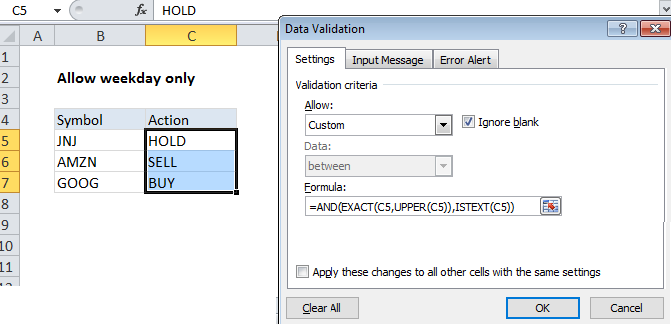## Excel Office

Excel How Tos, Tutorials, Tips & Tricks, Shortcuts

# Excel Data validation allow uppercase only

Using the example below, this tutorial shows how to create Excel Data validation to allow uppercase only.

## Formula

`=AND(EXACT(A1,UPPER(A1)),ISTEXT(A1))`

##Explanation

To allow a user to enter only uppercase TEXT,  you can use data validation with a custom formula based on the UPPER, EXACT, and AND functions.

In the example shown, the data validation applied to C5:C7 is:

`=AND(EXACT(C5,UPPER(C5)),ISTEXT(C5))`

### How this formula works

Data validation rules are triggered when a user adds or changes a cell value.

Worked Example:   How to count specific words in a cell in Excel

The UPPER function changes text values to uppercase, and the EXACT function performs a case-sensitive comparison.

The AND function takes multiple arguments (logical conditions) and returns TRUE only when all arguments return TRUE.

Worked Example:   Highlight dates in the next N days in Excel

The first logical condition compares the value input by the user to an uppercase version of the same value:

`EXACT(C5,UPPER(C5)`

The second logical condition tests that input to C5 is actually text

`ISTEXT(C5)`

If both conditions are TRUE, the AND function returns TRUE and input passes validation. If either condition is FALSE, AND returns FALSE and input fails data validation.

Worked Example:   Excel If, Nested If, And/Or Criteria Examples

Note:  Cell references in data validation formulas are relative to the upper left cell in the range selected when the validation rule is defined, in this case C5.